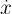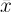# Complex¶

## Overview¶

XAD implements specialisations of std::complex for the XAD active data types AReal and FReal. They are are provided in the header XAD/Complex.hpp, along with all the mathematical operations defined in the standard.

Note that the complex header is not automatically included with XAD/XAD.hpp. Users must include it as needed.

template <typename T>
class
std::complex<AReal<T>>

Specialisation for adjoint mode data type (in std namespace).

template <typename T>
class
std::complex<FReal<T>>

Specialisation for forward mode data type (in std namespace).

## Members¶

All standard complex members are implemented.

Below are the non-standard additions and changes of the interface only, using the placeholder AReal<T> as a placeholder inner type. The same functions are also defined for FReal<T>.

XReal<T> &real()

This function returns a reference rather than a copy of the real part, to allow for easy access and adjusting of derivatives using derivative().

XReal<T> &imag()

Returns a reference rather than a copy.

const XReal<T> &real() const

Returns a reference rather than a copy.

const XReal<T> &imag() const

Returns a reference rather than a copy.

void setDerivative(const T &real_derivative,
const
T &imag_derivative = T()
)

Sets the derivatives (eitheror) for both the real and imaginary parts.

const
T &imag_derivative = T()
)

Alias for setDerivative()

std::complex<T> getDerivative() const

Gets the derivatives (eitheror) for both the real and imaginary parts, represented as a complex of the underlying (double) type.

Alias for getDerivative()

## Non-Member Functions¶

template <typename T>
std
::complex<T> derivative(const std::complex<AReal<T>> &z)

Returns the adjoints of the z variable, represented as a complex number of the underlying double type.

Note that since the return type is not a reference, setting derivatives should be done by using the member function template <typename T> std::complex<AReal<T> >::setDerivative() or using the real and imag member functions instead.

template <typename T>
std
::complex<T> derivative(const std::complex<FReal<T>> &z)

Returns the derivatives of the z variable, represented as a complex number of the underlying double type.

Note that since the return type is not a reference, setting derivatives should be done by using the member function template <typename T> std::complex<FReal<T> >::setDerivative() or using the real and imag member functions instead.

template <typename T>
std
::complex<T> value(const std::complex<AReal<T>> &z)

Returns the value of the z variable (underlying double type), represented as a complex number.

template <typename T>
std
::complex<T> value(const std::complex<FReal<T>> &z)

Returns the value of the z variable (underlying double type), represented as a complex number.

template <typename T>
AReal
<T> &real(std::complex<AReal<T>> &z)

template <typename T>
FReal
<T> &real(std::complex<FReal<T>> &z)

template <typename T>
AReal
<T> &imag(std::complex<AReal<T>> &z)